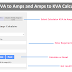In this conversion calculator, you can convert Amps into KVA(Kilo Volt-Ampere) and KVA into Amps. You can convert values for both Single Phase and Three Phase systems.

Amps to KVA and KVA to Amps Calculator
Click to Switch KVA to Amps
Click to Switch Amps to KVA

## KVA to Amps Calculator

Result in amps(A):
Click to Switch KVA to Amps
Click to Switch Amps to KVA

## Amps to kVA Calculator

Result in kilovolt-amps(KVA):

### How to Use This Calculator?

To use the above "KVA to Amps Calculator" and the "Amps to kVA Calculator," follow these steps:

KVA to Amps Calculator:

1. Select the Phase: Choose the phase type by selecting either "Single phase" or "Three phase" from the dropdown menu.

2. Enter Kilovolt-Amps (KVA): Input the value of Kilovolt-Amps (KVA) in the input box labeled "Enter kilovolt-amps(KVA)."

3. Select Voltage Type (For Three Phase Only - Optional): If you have chosen "Three phases" in the phase selection, another dropdown will appear. Select either "Line to line voltage" or "Line to neutral voltage" based on your requirement.

4. Enter Volts (V): Input the value of volts (V) in the input box labeled "Enter volts(V)."

5. Calculate: Click the "Calculate" button to perform the conversion.

6. View the Result: The result in amps (A) will be displayed in the "Result in amps(A):" input box.

7. Reset (Optional): If you want to clear all the input fields and results, you can click the "Reset" button.

Amps to kVA Calculator:

1. Select the Phase: Choose the phase type by selecting either "Single phase" or "Three phase" from the dropdown menu.

2. Enter Amps (A): Input the value of amps (A) in the input box labeled "Enter amps(A)."

3. Select Voltage Type (For Three Phase Only - Optional): If you have chosen "Three phase" in the phase selection, another dropdown will appear. Select either "Line to line voltage" or "Line to neutral voltage" based on your requirement.

4. Enter Volts (V): Input the value of volts (V) in the input box labeled "Enter volts(V)."

5. Calculate: Click the "Calculate" button to perform the conversion.

6. View the Result: The result in kilovolt-amps (KVA) will be displayed in the "Result in kilovolt-amps(KVA):" input box.

7. Reset (Optional): If you want to clear all the input fields and results, you can click the "Reset" button.

Note: For the "KVA to Amps Calculator," if you select "Three phase," additional options related to voltage type will appear. For the "Amps to kVA Calculator," the same applies if you select "Three phase."

### Formulas

Here are the formulas used in the above Calculator

kVA to Amps (Single-phase):

Amps = kVA * 1000 / Volts

kVA to Amps (Three-phase L-L):

Amps = kVA * 1000 / (Volts * √3)

kVA to Amps (Three-phase L-N):

Amps = kVA * 1000 / (Volts * 3)

Amps to kVA (Single-phase):

kVA = Amps * Volts / 1000

Amps to kVA (Three-phase L-L):

kVA = Amps * Volts * √3 / 1000

Amps to kVA (Three-phase L-N):

kVA = Amps * Volts * 3 / 1000

Thank you for visiting the Website. Keep visiting for more Updates.

Amps to KVA and KVA to Amps Conversion CalculatorReviewed by Author on August 04, 2023 Rating: 5